#### Value iteration

A value-iteration method can be derived by adapting the derivation that was applied to (10.33) to instead apply to (10.108). This leads to the dynamic programming recurrence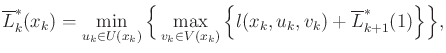(10.109)

which is analogous to (10.39). This can be used to iteratively compute a security plan for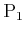. The security plan for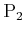can be computed using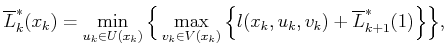(10.110)

which is the dynamic programming equation derived from (10.109).

Starting from the final stage,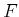, the upper and lower values are determined directly from the cost function: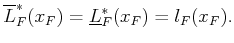(10.111)

Now compute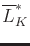and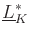. From every state,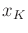, (10.110) and (10.111) are evaluated to determine whether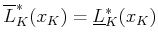. If this occurs, then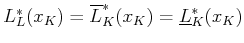is the value of the game fromat stage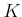. If it is determined that from any particular state,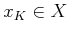, the upper and lower values are not equal, then there is no deterministic saddle point from. Furthermore, this will prevent the existence of deterministic saddle points from other states at earlier stages; these are encountered in later value iterations. Such problems are avoided by allowing randomized plans, but the optimization is more complicated because linear programming is repeatedly involved.

Suppose for now thatfor all. The value iterations proceed in the usual way from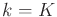down to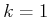. Again, suppose that at every stage,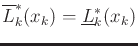for all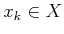. Note that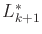can be written in the place of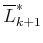and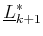in (10.110) and (10.111) because it is assumed that the upper and lower values coincide. If they do not, then the method fails because randomized plans are needed to obtain a randomized saddle point.

Once the resulting values are computed from each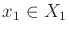, a security plan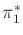foris defined for each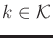andas any actionthat satisfies the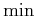in (10.110). A security plan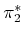is similarly defined forby applying any action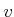that satisfies the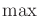in (10.111).

Now suppose that there exists no deterministic saddle point from one or more initial states. To avoid regret, randomized security plans must be developed. These follow by direct extension of the randomized security strategies from Section 9.3.3. The vectors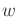and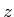will be used here to denote probability distributions over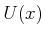and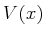, respectively. The probability vectors are selected from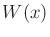and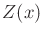, which correspond to the set of all probability distributions overand, respectively. For notational convenience, assume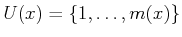and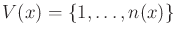, in which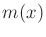and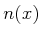are positive integers.

Recall (9.61) and (9.62), which defined the randomized upper and lower values of a single-stage game. This idea is generalized here to randomized upper and lower value of a sequential game. Their definitions are similar to (10.108) and (10.109), except that: 1) the alternating's and's are taken over probability distributions on the space of actions, and 2) the expected cost is used.

The dynamic programming principle can be applied to the randomized upper value to derive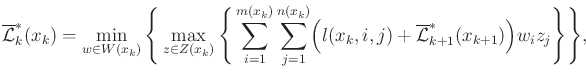(10.112)

in which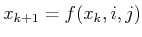. The randomized lower value is similarly obtained as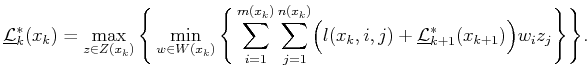(10.113)

In many games, the cost term may depend only on the state: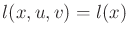for all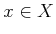,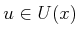and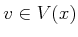. In this case, (10.113) and (10.114) simplify to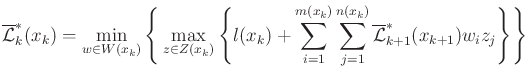(10.114)

and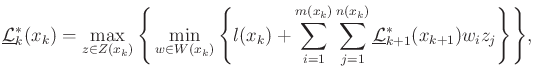(10.115)

which is similar to the simplification obtained in (10.46), in which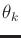was assumed not to appear in the cost term. The summations are essentially generalizations of (9.57) to the multiple-stage case. If desired, these could even be written as matrix multiplications, as was done in Section 9.3.3.

Value iteration can be performed over the equations above to obtain the randomized values of the sequential game. Since the upper and lower values are always the same, there is no need to check for discrepancies between the two. In practice, it is best in every evaluation of (10.113) and (10.114) (or their simpler forms) to first check whether a deterministic saddle exists from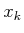. Whenever one does not exist, the linear programming problem formulated in Section 9.3.3 must be solved to determine the value and the best randomized plan for each player. This can be avoided if a deterministic saddle exists from the current state and stage.

Steven M LaValle 2020-08-14